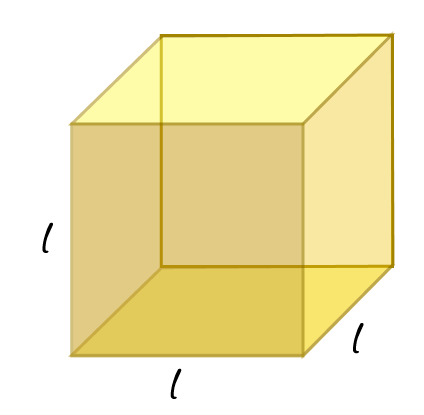# A cube is three dimensional figure as shown in Figure It has six faces and all of them are identical squares. The length of an edge of the cube is given by l. Find the formula for the total length of the edges of a cube.Solution:

Length of an edge of the cube = l

We know that

Number of edges in a cube = 12

Total length of the edges = Number of edges × length of an edge

=12l

The total length of edges of a cube= 12l(1)(0)